We’d like to understand how you use our websites in order to improve them. Register your interest.

# Existence of solutions for quasilinear elliptic equations with superlinear nonlinearities

## Abstract

Working in a weighted Sobolev space, a new result involving superlinear nonlinearities for a quasilinear elliptic boundary value problem in a domain in $R N$ is established. The proofs rely on the Galerkin method, Brouwer’s theorem and a new weighted compact Sobolev-type embedding theorem due to V.L. Shapiro.

MSC:35J25, 35J62, 65L60.

## 1 Introduction

Consider the following quasilinear elliptic problem:

${ M u = [ λ 1 u + f ( x , u ) ] ρ − G , x ∈ Ω , u ∈ H p , q , ρ 1 ( Ω , Γ ) ,$
(1.1)

where Ω is an open (possibly unbounded) set in $R N$ ($N≥1$), $λ 1$ is the first eigenvalue of $L$ ((2.3) below), and $M$ is a singular quasilinear elliptic operator defined by

$Mu=− ∑ i , j = 1 N D i [ p i 1 2 ( x ) p j 1 2 ( x ) σ i 1 2 ( u ) σ j 1 2 ( u ) b i j ( x ) D j u ] + b 0 σ 0 qu.$
(1.2)

The nonlinear part $f(x,u)$ in Eq. (1.1) satisfies certain superlinear conditions.

There have been many results for quasilinear elliptic equations under the conditions of which the nonlinearities satisfy sublinear or linear growth in a weighted Sobolev space. One can refer to .

However, there seem to be relatively few papers that consider the quasilinear elliptic equations with superlinearity, because the compactly embedding theorem cannot be obtained easily.

The aim of this paper is to obtain an existence result for problem (1.1). Our methods combine the Galerkin-type techniques, Brouwer’s fixed-point theorem, and a new compactly embedding theorem established by V.L. Shapiro in .

This paper is organized as follows. In Section 2, we introduce some necessary assumptions and main results. In Section 3, four fundamental lemmas are established. In Section 4, the proofs of the main results are given.

## 2 Assumptions and main results

In this section, we introduce some assumptions and give the main results in this paper.

Let $Γ⊂∂Ω$ be a fixed closed set (it may be the empty set) and $ρ(x), p i (x),q(x)∈ C 0 (Ω)$ be weight functions. $q(x)$ is nonnegative (maybe identically zero). Denote $p(x)$ as the vector function $( p 1 (x), p 2 (x),…, p N (x))$.

Consider the following pre-Hilbert spaces

$C ρ 0 (Ω)= { u ∈ C 0 ( Ω ) | ∫ Ω | u | 2 ρ < ∞ }$

with inner product $〈 u , v 〉 ρ = ∫ Ω uvρ$, $∀u,v∈ C ρ 0 (Ω)$, and

$C p , q , ρ 1 ( Ω , Γ ) = { u ∈ C 0 ( Ω ¯ ) ∩ C 2 ( Ω ) | u ( x ) = 0 , ∀ x ∈ Γ ; ∫ Ω [ ∑ i = 1 N | D i u | 2 p i + u 2 ( q + ρ ) ] < ∞ }$

with the inner product

$〈 u , v 〉 p , q , ρ = ∫ Ω ( ∑ i = 1 N p i D i u D i v + ( q + ρ ) u v )$
(2.1)

$∀u,v∈ C p , q , ρ 1 (Ω)$ where $D i u= ∂ u ∂ x i$, $i=1,…,N$. Let $L ρ 2 (Ω)$ be the Hilbert space obtained through the completion of $C ρ 0 (Ω)$ by using the method of Cauchy sequences with respect to the norm $∥ u ∥ ρ = 〈 u , u 〉 ρ 1 2$, and $H p , q , ρ 1 (Ω,Γ)$ be the completion of the space $C p , q , ρ 1 (Ω,Γ)$ with the norm $∥ u ∥ p , q , ρ = 〈 u , u 〉 p , q , ρ 1 2$. Similarly, we may have $L p i 2 (Ω)$ ($i=1,…,N$) and $L q 2 (Ω)$. Consequently, (2.1) may lead to

$〈 u , v 〉 p , q , ρ = ∑ i = 1 N 〈 D i u , D i v 〉 p i + 〈 u , v 〉 ρ + 〈 u , v 〉 q .$
(2.2)

Definition 2.1 For the quasilinear differential operator $M$, the two-form is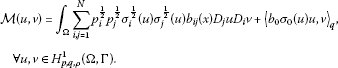For the linear differential operator,

$Lu=− ∑ i , j = 1 N D i [ p i 1 2 p j 1 2 a i j ( x ) D j u ] + a 0 qu,$
(2.3)

the two-form is

$L(u,v)= ∫ Ω ∑ i , j = 1 N p i 1 2 p j 1 2 a i j (x) D j u D i v+ 〈 a 0 u , v 〉 q ,∀u,v∈ H p , q , ρ 1 (Ω,Γ).$

Definition 2.2 $(Ω,Γ)$ is a simple-$V L$ region if the following conditions ($V L −1$)-($V L −5$) hold:

($V L −1$) There exists a complete orthonormal system ${ φ n } n = 1 ∞$ in $L ρ 2$. Also, $φ n ∈ H p , q , ρ 1 (Ω,Γ)∩ C 2 (Ω)$, n;

($V L −2$) There exists a sequence of eigenvalues ${ λ n } n = 1 ∞$, corresponding to the orthonormal sequence ${ φ n } n = 1 ∞$, and satisfying $0< λ 1 < λ 2 ≤ λ 3 ≤⋯≤ λ n →∞$ as $n→∞$, such that $L( φ n ,v)= λ n 〈 φ n , v 〉 ρ$, $∀v∈ H p , q , ρ 1 (Ω,Γ)$;

($V L −3$) $Ω= Ω 1 ×⋯× Ω N$, where $Ω i ⊂R$ is an open set for $i=1,…,N$;

($V L −4$) For each $p i (x)$ and $ρ(x)$ in ($V L −1$)-($V L −2$), associated with each $Ω i$ there are positive functions $p i ∗ (s), ρ i ∗ (s)∈ C 0 ( Ω i )$ satisfying $∫ Ω i [ p i ∗ (s)+ ρ i ∗ (s)]ds<∞$, and $ρ(x)= ρ 1 ∗ ( x 1 )⋯ ρ N ∗ ( x N )$, $p i (x)= ρ 1 ∗ ( x 1 )⋯ ρ i − 1 ∗ ( x i − 1 ) p i ∗ ( x i ) ρ i + 1 ∗ ( x i + 1 )⋯ ρ N ∗ ( x N )$, for $i=1,…,N$;

($V L −5$) For each $Ω i$, $p i ∗$, $ρ i ∗$ ($i=1,…,N$), there exists $h i ∈ C 0 ( Ω i )∩ L ρ i ∗ θ ( Ω i )$ for $2<θ<∞$ with the property

$|Φ(t)|≤ h i (t) ∥ Φ ∥ p i ∗ , ρ i ∗ ,∀Φ∈ C 1 ( Ω i ),∀t∈ Ω i ,$

where $∥ Φ ∥ p i ∗ , ρ i ∗ 2 = ∫ Ω i [ p i ∗ (t)| d Φ ( t ) d t | 2 + ρ i ∗ (t) Φ 2 (t)]dt$.

There are many examples to illustrate the Simple-$V L$ region. One can refer to  and .

Remark 2.1 From ($V L −3$) and ($V L −4$), it is easy to see that $ρ(x)$, $p i (x)$ are positive and

$∫ Ω ρ(x)dx<∞, ∫ Ω p i (x)dx<∞,i=1,…,N.$
(2.4)

Definition 2.3 $M$ is near-related to $L$ if the following condition holds:

We make the following assumptions concerning the operators $M$ and $L$: $a i j (x)$ ($i,j=1,…,N$) and $a 0 (x)$ satisfy (so do $b i j (x)$ and $b 0 (x)$):

(2.5)

It is assumed throughout the paper that $σ i (u)$ ($i=0,1,…,N$) meets:

($σ−1$) $σ i (u): H p , q , ρ 1 (Ω,Γ)→R$ is weakly sequentially continuous;

($σ−2$) $∃ η 0 , η 1 >0$, s.t. $η 0 ≤ σ i (u)≤ η 1$, $∀u∈ H p , q , ρ 1 (Ω,Γ)$.

$f(x,s)$ meets the following conditions:

($f−1$) $f(x,s)$ satisfies the Caratheodory conditions;

($f−2$) (superlinear growth condition) There exists θ with $2<θ< 2 N N − 1$, such that

where $h 0 (x)∈ L ρ θ ∗ (Ω)$. K is a nonnegative constant and $θ ∗ = θ θ − 1$.

($f−3$) There exists a nonnegative function $h ˜ 0 (x)∈ L ρ θ ∗ (Ω)$ and a constant $β ∗ >0$, such that

Remark 2.2 Observing that for $N=2$, $f(x,s)=−g(x)s|s | 5 3 − β ∗ s$, where $g(x)∈ C 0 (Ω)∩ L ∞ (Ω)$ is a positive function, and meets both ($f−2$) and ($f−3$).

Now we state our main results in this paper.

Theorem 2.1 Assume that $(Ω,Γ)$ is a simple-$V L$ region, the operator $M$ satisfies ($σ−1$)-($σ−2$), and is near-related to the operator $L$, (2.5) holds for both $M$ and $L$, f meets ($f−1$)-($f−3$), and $G∈ [ H p , q , ρ 1 ( Ω , Γ ) ] ′$ (the dual of $H p , q , ρ 1 (Ω,Γ)$). Then the problem (1.1) has at least one nontrivial weak solution, that is, there exists a $u ∗ ∈ H p , q , ρ 1 (Ω,Γ)$ such that

$M ( u ∗ , v ) = λ 1 〈 u ∗ , v 〉 ρ + ∫ Ω f ( x , u ∗ ) vρ−G(v)∀v∈ H p , q , ρ 1 (Ω,Γ).$

To derive out Theorem 2.1, we first discuss the problem in $S n$, which is the subspace of $H p , q , ρ 1 (Ω,Γ)$ spanned by $φ 1 ,…, φ n$. Then by virtue of the Galerkin method, the results will be extended to $H p , q , ρ 1 (Ω,Γ)$.

## 3 Fundamental lemmas

In this section, we introduce and establish four fundamental lemmas. Lemmas 3.1 and 3.2 give two useful embedding theorems. Lemma 3.3 constructs some approximation solutions in $S n$. Lemma 3.4 studies the properties of the approximation solutions.

Lemma 3.1 ()

Assume that $L$ is given by (2.3) and $(Ω,Γ)$ is a simple-$V L$ region. For $N≥2$, then $H p , q , ρ 1 (Ω,Γ)$ is compactly imbedded in $L ρ θ (Ω)$ for θ ($2<θ< 2 N N − 1$); for $N=1$, then $H p , q , ρ 1 (Ω,Γ)$ is compactly imbedded in $L ρ θ (Ω)$ for θ ($2<θ<∞$).

Lemma 3.2 ()

Assume that $L$ is given by (2.3) and $(Ω,Γ)$ is a simple-$V L$ region. Then $H p , q , ρ 1 (Ω,Γ)$ is compactly imbedded in $L ρ 2 (Ω)$.

Lemma 3.3 Let all the assumptions in Theorem 2.1 hold. Then for $n≥2$, there exists a $u n ∈ S n$ such that

$M( u n ,v)= ( λ 1 − 1 n ) 〈 u n , v 〉 ρ + ∫ Ω f(x, u n )vρ−G(v),∀v∈ S n .$
(3.1)

Proof For fixed n ($n≥2$) and $∀α=( α 1 ,…, α n )∈ R n$, set $u= ∑ k = 1 n α k φ k$. From simple-$V L$ conditions, we obtain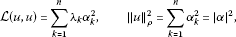(3.2)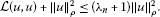(3.3)

From (3) and (4) of (2.5), we have

$L(u,u)+ ∥ u ∥ ρ 2 ≥ c 0 ∫ Ω ∑ i = 1 N p i | D i u | 2 + β 0 ∥ u ∥ q 2 + ∥ u ∥ ρ 2 ≥l ∥ u ∥ p , q , ρ 2 ,$
(3.4)

where $l=min{ c 0 , β 0 ,1}>0$. Combining (3.3) with (3.4), we get

$∥ u ∥ ρ 2 ≤ ∥ u ∥ p , q , ρ 2 ≤ λ n + 1 l ∥ u ∥ ρ 2 .$
(3.5)

For $m≥2$, a positive integer, we put

$f m (x,s)={ f ( x , m ) , m ≤ s ; f ( x , s ) , − m ≤ s ≤ m ; f ( x , − m ) , s ≤ − m .$
(3.6)

Note from ($f−2$) that $| f m (x,s)|≤ h 0 (x)+K|m | θ − 1$ for $∀s∈R$, a.e. $x∈Ω$. Also, from $h 0 (x)∈ L ρ θ ∗$, the Hölder inequality, Minkowski inequality, and (2.4), for $∀v∈ S n$, we get

$∫ Ω | f m (x,u)vρ|≤ ∥ f m ( x , u ) ∥ L ρ θ ∗ ∥ v ∥ L ρ θ ≤ T m ∥ v ∥ L ρ θ ,$
(3.7)

where $T m$ is a positive constant depending on m.

The remaining proof is separated into two parts. The first part is to prove the claim (3.8) for $f m (x,s)$. The second part is to get the conclusion by leaving $m→∞$ based on (3.8).

Part 1. Fix m ($m≥2$). To show there exists $u n , m ∗$ such that

$M ( u n , m ∗ , v ) = ( λ 1 − 1 n ) 〈 u n , m ∗ , v 〉 ρ + ∫ Ω f m ( x , u n , m ∗ ) vρ−G(v),∀v∈ S n ,$
(3.8)

we set

$F k (α)=M(u, φ k )− ( λ 1 − 1 n ) 〈 u , φ k 〉 ρ − ∫ Ω f m (x,u) φ k ρ+G( φ k ),k=1,…,n.$

It is clear that $∑ k = 1 n F k (α) α k =I(α)+II(α)$, where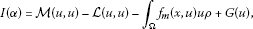(3.9)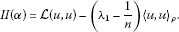(3.10)

For (3.9), observing the fact that the operator $M$ is near-related to $L,G∈ [ H p , q , ρ 1 ( Ω , Γ ) ] ′$, (3.2), (3.5), (3.7), and Lemma 3.1, we conclude that

$lim | α | → ∞ M ( u , u ) − L ( u , u ) | α | 2 =0, lim | α | → ∞ | ∫ Ω f m ( x , u ) u ρ | | α | 2 =0, lim | α | → ∞ | G ( u ) | | α | 2 =0,$

and

$lim | α | → ∞ | I ( α ) | | α | 2 =0.$

For (3.10), by (3.2), we obtain

$II(α)= ∑ k = 1 n ( λ k − λ 1 + 1 n ) α k 2 ≥ 1 n | α | 2 .$
(3.11)

Consequently, $∑ k = 1 n F k (α) α k ≥ | α | 2 2 n$ where $|α|≥ s 0$ (here $s 0$ is a large enough constant). By virtue of the generalized Brouwer’s theorem , there exists $γ n , m =( γ n , m ( 1 ) ,…, γ n , m ( n ) )$, such that $F k ( γ n , m )=0$, $k=1,…,n$. Taking $u n , m ∗ = ∑ k = 1 n γ n , m ( k ) φ k$, then (3.8) holds.

Part 2. We claim that ${ ∥ u n , m ∗ ∥ ρ } m = 2 ∞$ (n fixed) is uniformly bounded according to m.

Arguing by contradiction, without loss of generality, suppose that

$lim m → ∞ ∥ u n , m ∗ ∥ ρ =∞.$
(3.12)

Taking $v= u n , m ∗$ in (3.8),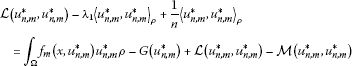(3.13)

holds, that is,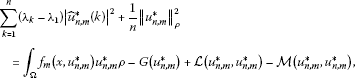(3.14)

where $u ˆ n , m ∗ (k)= 〈 φ k , u n , m ∗ 〉 ρ$.

On the other hand, using ($f−3$), for $s≥m$, we have

(3.15)

Similarly, we can also obtain the same conclusion where $−m≤s≤m$ or $s≤−m$. As a result,

(3.16)

(3.14) and (3.16) imply that

$1 n ∥ u n , m ∗ ∥ ρ 2 ≤ ∥ h ˜ 0 ∥ L ρ θ ∗ ∥ u n , m ∗ ∥ L ρ θ +|G ( u n , m ∗ ) |+L ( u n , m ∗ , u n , m ∗ ) −M ( u n , m ∗ , u n , m ∗ ) .$
(3.17)

Dividing both sides of (3.17) by $∥ u n , m ∗ ∥ ρ 2$ and leaving $m→∞$, we obtain from the fact that $M$ is near-related to $L,G∈ [ H p , q , ρ 1 ( Ω , Γ ) ] ′$, $h ˜ 0 (x)∈ L ρ θ ∗ (Ω)$, and (3.5) together with Lemma 3.1 that $1 n ≤0$. However, n is a positive integer. So, we have arrived at a contradiction. (3.12) does not hold. Then

$∃ K 1 >0, ∥ u n , m ∗ ∥ ρ ≤ K 1 ,∀m≥2.$
(3.18)

(3.5) and (3.18) imply that there is a subsequence of ${ u n , m ∗ } m = 2 ∞$ (for ease of notation take the full sequence) and a $u n ∈ S n$  such that

(3.19)

Therefore, from (3.19), we obtain

$lim m → ∞ M ( u n , m ∗ , v ) =M( u n ,v),∀v∈ S n .$
(3.20)

And recall Lemma 3.1 that

$lim m → ∞ ∫ Ω | u n , m ∗ − u n | θ ρ=0.$
(3.21)

Moreover, there exists a $W(x)∈ L ρ θ$ and a subsequence ${ u n , m j ∗ } j = 1 ∞ ⊂ { u n , m ∗ } m = 2 ∞$ such that $| u n , m j ∗ (x)|≤W(x)$, a.e. $x∈Ω$ for j.

Since by virtue of the Hölder inequality, ($f−1$), ($f−2$) and the Lebesgue dominated convergence theorem, we get

$lim j → ∞ ∫ Ω f m j ( x , u n , m j ∗ ) vρ= ∫ Ω f(x, u n )vρ,∀v∈ S n .$

Now replacing m by $m j$ in (3.8) and taking the limit as $j→∞$ on both sides of the equation, we consequently obtain that (3.1) holds and Lemma 3.3 is completed. □

Lemma 3.4 Let all the assumptions in Theorem 2.1 hold. Then the sequence ${ u n }$ obtained in Lemma 3.3 is uniformly bounded in $H p , q , ρ 1 (Ω,Γ)$.

Proof For ${ u n } n = 2 ∞ ⊂ S n$ in Lemma 3.3, set $u n = ∑ k = 1 n u ˆ n (k) φ k$ where $u ˆ n (k)= 〈 φ k , u n 〉 ρ$.

We suppose that Lemma 3.4 is false. Without loss of generality, suppose that

$lim n → ∞ ∥ u n ∥ p , q , ρ =∞.$
(3.22)

To lead to a contradiction, taking $v= u n$ in (3.1), then

$M( u n , u n )= ( λ 1 − 1 n ) 〈 u n , u n 〉 ρ + ∫ Ω f(x, u n ) u n ρ−G( u n ).$
(3.23)

And we can get

$1 n ∥ u n ∥ ρ 2 ≤ ∫ Ω f(x, u n ) u n ρ+|G( u n )|+L( u n , u n )−M( u n , u n ).$
(3.24)

In view of $G∈ [ H p , q , ρ 1 ( Ω , Γ ) ] ′$, ($f−3$), and (3.24),

$( 1 n + β ∗ ) ∥ u n ∥ ρ 2 ≤ ∥ h ˜ 0 ∥ L ρ θ ∗ ∥ u n ∥ L ρ θ +|G( u n )|+L( u n , u n )−M( u n , u n ).$
(3.25)

Dividing both sides of (3.25) by $∥ u ∥ p , q , ρ 2$ and leaving $n→∞$, from the fact that $M$ is near-related to $L, h ˜ 0 (x)∈ L ρ θ ∗$ and Lemma 3.1, we get

$lim n → ∞ ∥ u n ∥ ρ ∥ u n ∥ p , q , ρ =0.$
(3.26)

Apply (3) and (4) of (2.5) in conjunction with ($σ−2$) to show

$M( u n , u n )≥ c 1 η 0 ∫ Ω ∑ i = 1 N p i | D i u n | 2 + β 1 η 0 ∥ u n ∥ q 2 ,$
(3.27)

also, (1) and (2) of (2.5) to show

$L( u n , u n )≤ K 2 ∫ Ω ∑ i = 1 N p i | D i u n | 2 + K 3 ∥ u n ∥ q 2 ,$
(3.28)

where $K 2$ and $K 3$ are positive constants. Setting $c 2 =min{ c 1 η 0 , β 1 η 0 }$ and $c 3 =max{ K 2 , K 3 }$, it is obvious $c 2 , c 3 >0$. By (3.27) and (3.28), we conclude that

$c 2 c 3 L( u n , u n )≤M( u n , u n ).$
(3.29)

Using (3.29) and ($f−3$), from (3.25), we obtain

$c 2 c 3 L( u n , u n )+ β ∗ ∥ u n ∥ ρ 2 ≤ λ 1 ∥ u n ∥ ρ 2 + ∥ h ˜ 0 ∥ L ρ θ ∗ ∥ u n ∥ L ρ θ +|G( u n )|.$
(3.30)

From (3.4), we get $L( u n , u n )+ ∥ u n ∥ ρ 2 ≥l ∥ u n ∥ p , q , ρ 2$. Set $c 4 =min{ c 2 c 3 , β ∗ }$. It is easy to obtain

$c 4 l ∥ u n ∥ p , q , ρ 2 ≤ λ 1 ∥ u n ∥ ρ 2 + ∥ h ˜ 0 ∥ L ρ θ ∗ ∥ u n ∥ L ρ θ +|G( u n )|.$
(3.31)

Dividing both sides of (3.31) by $∥ u ∥ p , q , ρ 2$ and leaving $n→∞$, by (3.26), we get

$c 4 l≤0.$
(3.32)

So, we have arrived at a contradiction. Thus, there holds

(3.33)

Lemma 3.4 is completed. □

## 4 Proof of Theorem 2.1

Proof Since $H p , q , ρ 1 (Ω,Γ)$ is a separable Hilbert space, from Lemma 3.1 and Lemma 3.2, we conclude that there exist a subsequence of ${ u n } n = 2 ∞$ (which for ease of notation we take the full sequence) and a function $u ∗ ∈ H p , q , ρ 1 (Ω,Γ)$ with the following properties :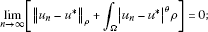(4.1)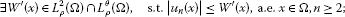(4.2)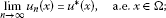(4.3)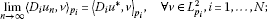(4.4)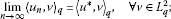(4.5)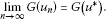(4.6)

Let $v J ∈ S J$ where $J≥2$ is a fixed but arbitrary positive integer. In fact, for $n≥J$, we have

$M ( u n , v J ) − M ( u ∗ , v J ) = ∫ Ω ∑ i , j = 1 N { p i 1 2 p j 1 2 σ i 1 2 ( u n ) σ j 1 2 ( u n ) b i j ( x ) D j ( u n − u ∗ ) D i v J + p i 1 2 p j 1 2 [ σ i 1 2 ( u n ) σ j 1 2 ( u n ) − σ i 1 2 ( u ∗ ) σ j 1 2 ( u ∗ ) ] b i j ( x ) D j u ∗ D i v J } + 〈 b 0 [ σ 0 ( u n ) u n − σ 0 ( u ∗ ) u ∗ ] , v J 〉 q .$
(4.7)

Observing (1) of (2.5), ($σ−1$)-($σ−2$), (4.3)-(4.5) and $D i v J ∈ L p i 2$, we have

$lim n → ∞ M( u n , v J )=M ( u ∗ , v J ) .$
(4.8)

On the other hand, applying ($f−1$), ($f−2$), (4.2), (4.3), the Hölder inequality, and the Lebesgue dominated convergence theorem, we obtain that

(4.9)

Also, by (3.1), we have

$M( u n , v J )= ( λ 1 − 1 n ) 〈 u n , v J 〉 ρ + ∫ Ω f(x, u n ) v J ρ−G( v J ).$
(4.10)

For (4.10), leaving $n→∞$, from (4.1), (4.8), and (4.9), we have

$M ( u ∗ , v J ) = λ 1 〈 u ∗ , v J 〉 ρ + ∫ Ω f ( x , u ∗ ) v J ρ−G( v J ).$
(4.11)

Next, given $v∈ H p , q , ρ 1 (Ω,Γ)$, we define a projection $P J : H p , q , ρ 1 (Ω,Γ)⟶ S J$, that is,

$P J v= ∑ k = 1 J v ˆ (k) φ k ∈ S J ,$
(4.12)

where $v ˆ (k)= 〈 φ k , v 〉 ρ$. It is easy to get $lim j → ∞ ∥ P J v − v ∥ p , q , ρ =0$. As a result, there hold

${ lim J → ∞ M ( u ∗ , P J v ) = M ( u ∗ , v ) , lim J → ∞ 〈 u ∗ , P J v 〉 ρ = 〈 u ∗ , v 〉 ρ , lim J → ∞ ∫ Ω f ( x , u ∗ ) P J v ρ = ∫ Ω f ( x , u ∗ ) v ρ , lim J → ∞ G ( P J v ) = G ( v ) .$
(4.13)

Replacing $v J$ by $P J v$ in (4.11), passing to the limit as $J→∞$ on both sides, and using (4.13), we can obtain

$M ( u ∗ , v ) = λ 1 〈 u ∗ , v 〉 ρ + ∫ Ω f ( x , u ∗ ) vρ−G(v),∀v∈ H p , q , ρ 1 (Ω,Γ).$

Hence, the proof of Theorem 2.1 is complete. □

## References

1. 1.

Berestycki H, Figueredo DG: Double resonance in semilinear elliptic problems. Commun. Partial Differ. Equ. 1981, 6(1):91-120. 10.1080/03605308108820172

2. 2.

Rumbos A, Shapiro VL: Jumping nonlinearities and weighted Sobolev spaces. J. Differ. Equ. 2005, 214: 326-357. 10.1016/j.jde.2004.11.005

3. 3.

Rumbos A: A semilinear elliptic boundary value problem at resonance where the nonlinearity may grow linearly. Nonlinear Anal. TMA 1991, 16: 1159-1168. 10.1016/0362-546X(91)90203-D

4. 4.

Lefton L, Shapiro VL: Resonance and quasilinear parabolic differential equations. J. Differ. Equ. 1993, 101: 148-177. 10.1006/jdeq.1993.1009

5. 5.

Shapiro VL: Resonance, distributions and semilinear elliptic partial differential equations. Nonlinear Anal. TMA 1984, 8: 857-871. 10.1016/0362-546X(84)90108-1

6. 6.

Jia G, Zhao Q: Existence results in weighted Sobolev spaces for some singular quasilinear elliptic equations. Acta Appl. Math. 2010, 109: 599-607. 10.1007/s10440-008-9336-x

7. 7.

Shapiro VL Memoirs of the American Mathematical Society 726. In Singular Quasilinearity and Higher Eigenvalues. Am. Math. Soc., Providence; 2001.

8. 8.

Shapiro VL: Special functions and singular quasilinear partial differential equations. SIAM J. Math. Anal. 1991, 22: 1411-1429. 10.1137/0522090

9. 9.

Kesavan S: Topics in Functional Analysis and Applications. Wiley, New York; 1989.

10. 10.

Xuan BJ: Variational Methods. University of Science and Technology of China Press, Hefei; 2006.

## Acknowledgements

The authors express their sincere thanks to the referees for their valuable suggestions. This work was supported financially by the National Natural Science Foundation of China (11171220).

## Author information

Authors

### Corresponding author

Correspondence to Jia Gao.

### Competing interests

The authors declare that they have no competing interests.

### Authors’ contributions

The paper is the result of joint work of all authors who contributed equally to the final version of the paper. All authors read and approved the final manuscript.

## Rights and permissions

Reprints and Permissions

Gao, J., Lina, H. & Xiaojuan, Z. Existence of solutions for quasilinear elliptic equations with superlinear nonlinearities. Bound Value Probl 2012, 90 (2012). https://doi.org/10.1186/1687-2770-2012-90